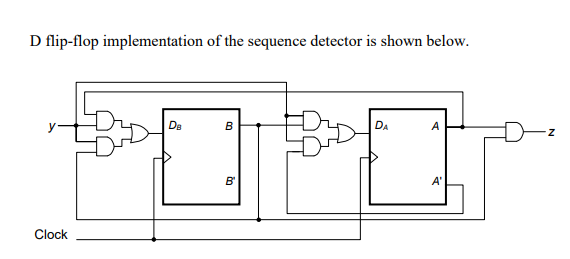Sequential Circuits - Flip Flops - 15Which of the following pattern is detected by the above circuit?

(A) It detects if a string has three or more consecutive ones

(B) It detects  if a string has four or more consecutive ones

(C) It detects  if a string has two or more consecutive ones

(D) It detects if a  string has a pattern 1010asked May 19 in Digital
reshown May 20

Please go through the explanation...answered May 21 by (31,410 points)
When BA is 00 and y is 0 o/p is 1. So ans should be C.Why A?
why o/p is 1 ?  can you elaborate it .
Sorry BA is 11 and y is 0 then o/p is 1.. so it also detects two ones,right?
A is correct oneanswered May 20 by (24,690 points)
+1 vote
@getgatebook

Please provide explanation for this question.answered May 20 by (11,660 points)
Sure. We will do it by tomorrow.
Sir there are many questions which need your explanation.
please try to explain necessarily those questions which are not yet marked as best answer.
just concentrate on first ff y=1 and let all others be 0 so first flip flop will propogate 0

now if A=0 then A'=1 and second gate for second flip flop will be on so it will now propogate 1

this was first y=1

from now on first ff will easily propogate 1 and same is for second because of upper and gate of both will always be high now see the inputs of last and gate it will get on after second pass

so it can detect 2 or more sequence of 1answered Jun 2 by (170 points)